# 1. 香农编码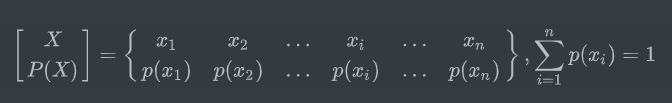1. 将信源符号按概率从大到小排列，令$p(x_1)\geq p(x_2)\geq \dots \geq p(x_n)$

2. 令$p(x_0)=0$，用$p_a(x_j),j=i+1$表示第j个码字的累加概率，则：
$$p_a(x_j)= \sum_{i=0}^{j-1}{p(x_i)},j=1,2,\dots,n$$

3. 确定满足下列不等式的整数$l_i$，并令$l_i$为第i个码字的长度
$$-\log{p(x_i)}\leq l_i< -\log{p(x_i)}+1$$

4. 将$p_a(x_j)$用二进制表示，并取小数点后$l_i$位作为符号$x_i$的编码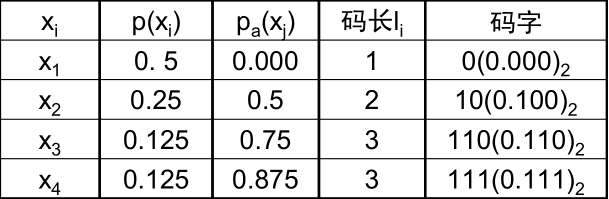• 一般情况下编码效率不是很高，编出来的不是最佳码
• 特殊情况下，才有高的编码效率
• 求码长时，左边的等号成立，右边的不成立

# 2. 费诺编码

1. 将概率从大到小排列，令$p(x_1)\geq p(x_2)\geq \dots \geq p(x_n)$
2. 按编码进制数将概率分组，使每组概率尽可能接近或相等，编m进制分m组
3. 给每组分配一个码元
4. 将每一组再按同样原则划分，重复步骤2和3，直到每个组只剩下一个信源符号为止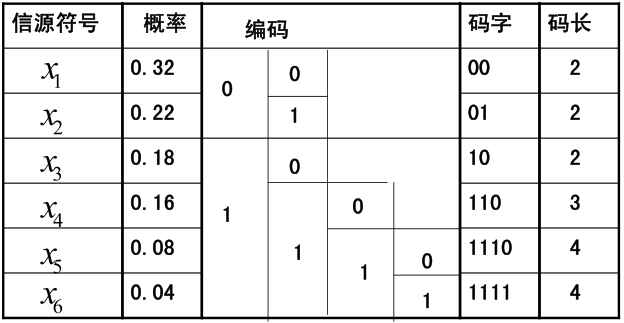• 费诺码适合于每次分组概率都很接近的信源，特别是对每次分组概率都相等的信源进行编码时，可达到理想的编码效率

• 费诺码是即时码
• 概率大的信源符号能对应码长较短的码字
• 费诺码不一定是最佳码：不一定能使短码得到充分利用

# 3. 霍夫曼编码

1. 将概率按从大到小的顺序排列，令$p(x_1)\geq p(x_2)\geq \dots \geq p(x_n)$
2. 给两个概率最小的信源符号$p(x_{n-1})$和$p(x_n)$各分配一个码位“0”和“1”，将这两个信源符号合并成一个新符号，并用这两个最小概率之和作为新符号的概率，结果得到一个只包含(n-1)个符号的新信源，称为信源的第一次缩减信源，用S1表示
3. 将缩减信源S1仍按概率从大到小排列，重复步骤2，得到只含(n-2)个符号的缩减信源
4. 重复上述步骤，直至缩减信源只剩两个符号为止，此时所剩的两个符号的概率之和为1，然后从最后一级缩减信源开始，依编码路径向前返回，就得到各信源符号对应的码字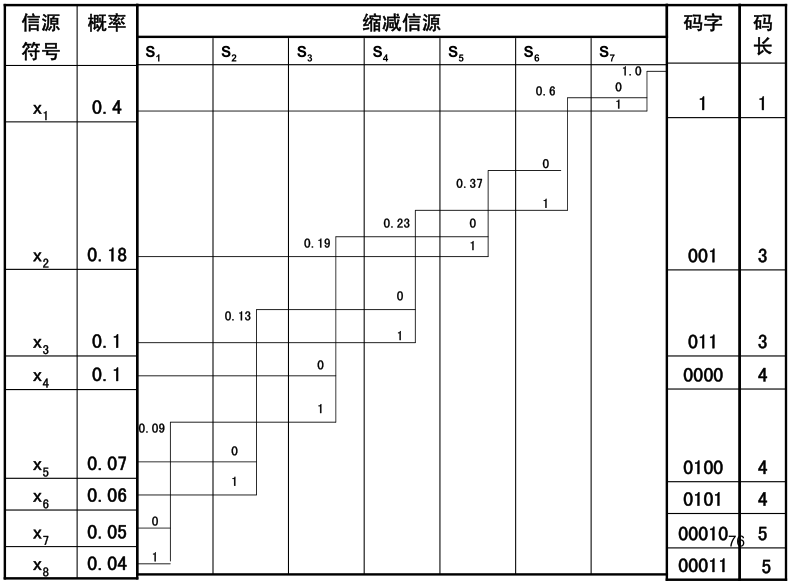• 霍夫曼编码不唯一：每次对两个概率最小的符号分配“0，1”是任意的，合并后新符号概率与其他符号概率相等时这几个符号的次序也是可以任意排列的
• 合并后的新符号尽量排在靠前的位置，这样码长的方差比较小
• 合并后的新符号重复编码次数减少，使短码得到充分利用
• r元码，信源S的符号个数q必须满足：$q=n(r-1)+r$（n为非负整数，r-1为每次缩减所减少的信源符号个数）。对于二元码，$q=n+2$，n可为任意非负整数
• 对于不满足条件的r元码，可假设一些信源符号: $S_{q+1},S_{q+2},\dots,S_{q+t}$作为虚拟符号，并令它们的概率为0，使得$q=n(r-1)+r$成立，这样处理后的r元霍夫曼编码可充分利用短码，一定是紧致码

• 是分组码
• 是唯一可译码
• 是即时码

# 4. 香农-费诺-埃利斯码

1. 设信源符号集$A={a_1,a_2,\dots,a_q}$，并设所有$P(a_i)>0$，计算累积分布函数：$F(s)=\sum_{a\leq s}{P(a_i)}$

2. 计算修正的累积分布函数：
$$\overline{F}(s)=\overline{F}(a_k)=\sum_{i=1}^{k-1}{P(a_i)+{1\over 2}P(a_k)}$$

3. 计算码长：$l(s)=\lceil \log{1\over p(s)}\rceil+1$

4. 将修正后的累积分布函数用二进制表示，并取小数点后$l(s)$位作为符号$x_i$的编码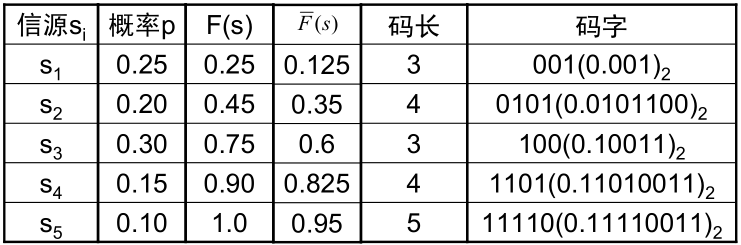# 5. 算术编码

• 香农-费诺-埃利斯码不是最佳码，但由它可拓宽得到一种算数码
• 不同于霍夫曼码，是一种非分组码，其编码和译码都是计算效率高的码
• 从全序列出发，考虑符号之间的依赖关系
• 主要应用于计算机数据交换或文本存储，音视频数据的压缩

# 6. 游程编码

• 游程（RL）：数字序列中连续出现相同符号的一段
• 游程编码（RLC）：将游程序列映射成游程长度和对应符号序列的位置的标志序列
• 连续的“0”的长度为L(0)，连续的“1”的长度为L(1)

• 对于随机序列，游程长度是随机的
• 游程变换：是一一对应的变换，也是可逆变换
• 规定二元序列总是从0开始
• 游程变换减弱了原序列符号间的相关性
• 游程将二元序列变换成了多元序列
• 编码方法：
• 首先测定0和1的游程长度的概率分布，即以游程长度为元素，构造一个新的信源
• 对新的信源（游程序列）进行霍夫曼编码
• 截断处理：
• 选取一个适当的n，将游程长度定为一共有2^n^个，所有游程大于2^n^的，都用游程为2^n^的这个码字来处理
• 将这2^n^个游程按概率大小进行霍夫曼编码，得到相应的码字，也就获得游程为2^n^的这个码字C
• 对所有大于2^n^以上的游程进行编码，所有大于2^n^、小于2^n+1^的，就用码字C之后加一个n位的自然码A构成对应的码字。A代表余数，用以区分$2^n-2^{n+1}$之间的不同长度
• 二元独立序列游程长度的熵、平均游程长度：
• $p[L(0)]=p_0^{L(0)-1}p_1$
• $p[L(1)]=p_1^{L(1)-1}p_0$
• $H[L(0)]={H(p_0)\over p_1}, l_0={1\over p_1}$
• $H[L(1)]={H(p_1)\over p_0},l_1={1\over P_0}$
• 二元独立序列的熵：$H(X)=H(p_0)=H(p_1)$

# 7. MH编码

• MH编码是用于黑白二值文件传真的数据压缩，它们是黑白二值的，即信源是二元信源q=2
• MH码的码字分为终端吗和组合码
• 编码规则
1. 游程长度在0~63之间时，码字直接用相应的终端码表示
2. 游程长度在64~1728，用一个组合码加上一个终端码作为相应码字
3. 规定每行都从白游程开始，若实际出现黑游程，则在行首加上长度为零的白游程字，每行结束时用一个结束码（EOL）作标记
4. 每页文件开始第一个数据前加一个结束码，每页结尾连续使用6个结束码表示结尾
5. 译码时，每一行码都应恢复出1728个像素，否则有错
6. 为了在传输时可实现同步操作，规定T为每个编码行的最小传输时间，若编码行传输时间小于T，则在结束码之前填上足够多的“0”码元（称填充码）

上一篇使用Hexo写博客的一些小技巧

2020-08-04计算机组成原理之寻址方式

2020-05-12
目录